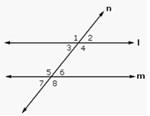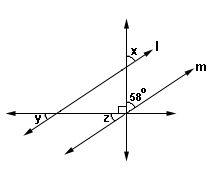Definition Of Corresponding Angles

Corresponding Angles are two congruent angles, both lying on the same side of the transversal and situated the same way on two different parallel lines.

In the figure shown, l and m are two parallel lines cut by the transversal n. Angles 2 & 6, 4 & 8, 1 & 5, and 3 & 7 are corresponding angles.Solved Example on Corresponding Angles

Ques: How many pairs of corresponding angles are formed when two parallel lines are cut by a transversal?A. 4
B. 6
C. 8
D. 2